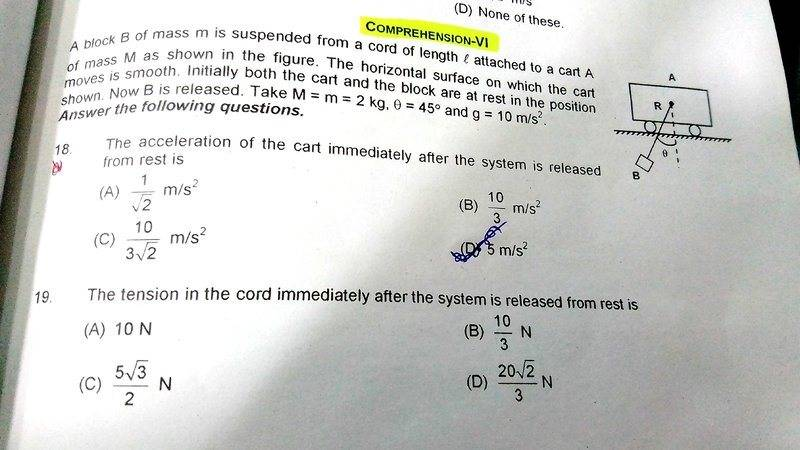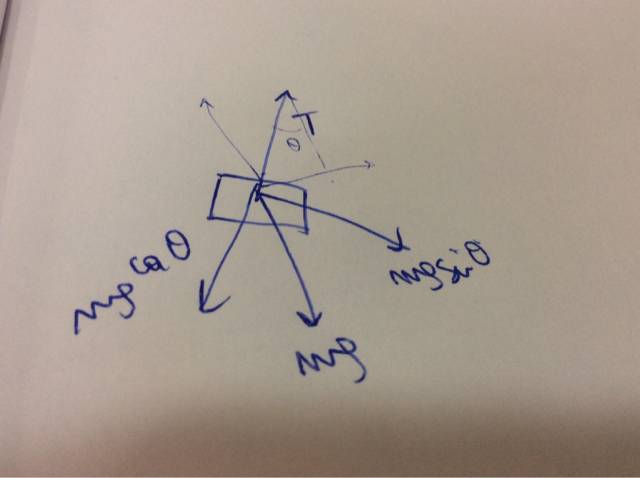# Acceleration of a cart immediately after the system is released

## Homework Statement

:
A block B of mass m is suspended from a cord of length l attached to cart A of mass M as shown in the figure. The horizontal surface on which the cart moves is smooth. Initially the cart and the block are at rest in the position shown. Now the block is released. Take M=2kg, θ=45⁰ and g=10m/s².
Acceleration of the cart immediately after the system is released from rest and tension in the cord developed immediately is?

## Homework Equations

: [/B]Balancing components of forces.

## The Attempt at a Solution

; [/B]
so as soon as the system is released from rest, tension in the chord acts, and I think that is because of mgCosθ . So tension should be 20cos45⁰ which is not the answer.
For horizontal acceleration of cart, I’m confused. I believe tension force gives the horizontal component of force which is responsible for accelertion of the cart. But since no external force is acting on the system, then centre of mass should not move, which means it will have acceleration in opposite direction of acceleration of block B. After this i dont know what to do.
Sorry for poor quality of the image.tnich
Homework Helper

## Homework Statement

:
A block B of mass m is suspended from a cord of length l attached to cart A of mass M as shown in the figure. The horizontal surface on which the cart moves is smooth. Initially the cart and the block are at rest in the position shown. Now the block is released. Take M=2kg, θ=45⁰ and g=10m/s².
Acceleration of the cart immediately after the system is released from rest and tension in the cord developed immediately is?

## Homework Equations

: [/B]Balancing components of forces.

## The Attempt at a Solution

; [/B]
so as soon as the system is released from rest, tension in the chord acts, and I think that is because of mgCosθ . So tension should be 20cos45⁰ which is not the answer.
For horizontal acceleration of cart, I’m confused. I believe tension force gives the horizontal component of force which is responsible for accelertion of the cart. But since no external force is acting on the system, then centre of mass should not move, which means it will have acceleration in opposite direction of acceleration of block B. After this i dont know what to do.
Sorry for poor quality of the image.View attachment 225607
The center of mass should not accelerate in the opposite direction from the block. If it is not moving, it will not accelerate at all.
Given your expression for the tension on the chord, what is the horizontal component of the force? What acceleration does it cause for the block?
How does this compare with the horizontal acceleration of the pendulum mass? What is the effect on the center of mass?

haruspex
Science Advisor
Homework Helper
Gold Member
2020 Award
So tension should be 20cos45⁰
If that were the case, what would be the vertical acceleration of block B?

If that were the case, what would be the vertical acceleration of block B?

That is the reason I’m confused. Vertical acceleration of block B is g? or is there some centripetal force acting whose vertical component gets added up?

The center of mass should not accelerate in the opposite direction from the block. If it is not moving, it will not accelerate at all.
Given your expression for the tension on the chord, what is the horizontal component of the force? What acceleration does it cause for the block?
How does this compare with the horizontal acceleration of the pendulum mass? What is the effect on the center of mass?

By “it” i meant the cart, not the centre of mass. Can you please rephrase your question a bit?[emoji28]

tnich
Homework Helper
By “it” i meant the cart, not the centre of mass. Can you please rephrase your question a bit?[emoji28]
OK. What is the horizontal component of the tension on the cord?

OK. What is the horizontal component of the tension on the cord?

mgCosθSinθ?Sorry for my poor handwriting.
But I think according to the FBD i have sketched, Tcosθ=mg. and not T=mgCosθ

#### Attachments

tnich
Homework Helper
mgCosθSinθ?View attachment 225641
Sorry for my poor handwriting.
But I think according to the FBD i have sketched, Tcosθ=mg. and not T=mgCosθ
No, you were right the first time, and you are correct on the horizontal component of the tension. So what is the acceleration of the block?

No, you were right the first time, and you are correct on the horizontal component of the tension. So what is the acceleration of the block?

mgCosθSinθ/M=10/2=5m/s² but that is not the answer, the answer given is 10/3

haruspex
Science Advisor
Homework Helper
Gold Member
2020 Award
But I think according to the FBD i have sketched, Tcosθ=mg. and not T=mgCosθ
Neither. T=mgCosθ would be correct if the cart were fixed, but the cart's acceleration reduces the tension.

Let the cart's acceleration be a to the left, while suspended mass has accelerations b to the right (in the lab frame) and c down.
What equations can you write relating these to the tension?
What equation in these expresses the fact that the string has constant length?

Neither. T=mgCosθ would be correct if the cart were fixed, but the cart's acceleration reduces the tension.

Let the cart's acceleration be a to the left, while suspended mass has accelerations b to the right (in the lab frame) and c down.
What equations can you write relating these to the tension?
What equation in these expresses the fact that the string has constant length?
i guess mc=mg-TCosθ
mb=Tsinθ
since centre of mass should not accelerate, i guess a=b

haruspex
Science Advisor
Homework Helper
Gold Member
2020 Award
i guess mc=mg-TCosθ
mb=Tsinθ
since centre of mass should not accelerate, i guess a=b
Right. What about my last question in post #10?

Right. What about my last question in post #10?

By “string has constant length” do you mean the string remains taut and does not get slack at any point of time or do you mean it is unstretchable?
Can you give a little more hint?

haruspex
Science Advisor
Homework Helper
Gold Member
2020 Award
By “string has constant length” do you mean the string remains taut and does not get slack at any point of time or do you mean it is unstretchable?
Both.
Can you give a little more hint?
If two accelerating objects stay a constant distance apart, what can you say about their accelerations in a certain direction?

Both.

If two accelerating objects stay a constant distance apart, what can you say about their accelerations in a certain direction?

They have equal component of acceleration in that direction? Equal in magnitude. not sure of the sign. should have same sign as well. if they stay at constant distance apart, then relative acceleration should be zero in the direction tangential to the string ? But which of the three equations I wrote is expressed here? a=b ? but then relative acceleration of the block and the cart will not be zero[emoji27]

haruspex
Science Advisor
Homework Helper
Gold Member
2020 Award
relative acceleration should be zero in the direction tangential to the string ?
Right.
First, find the relative acceleration of the two masses as horizontal and vertical components.